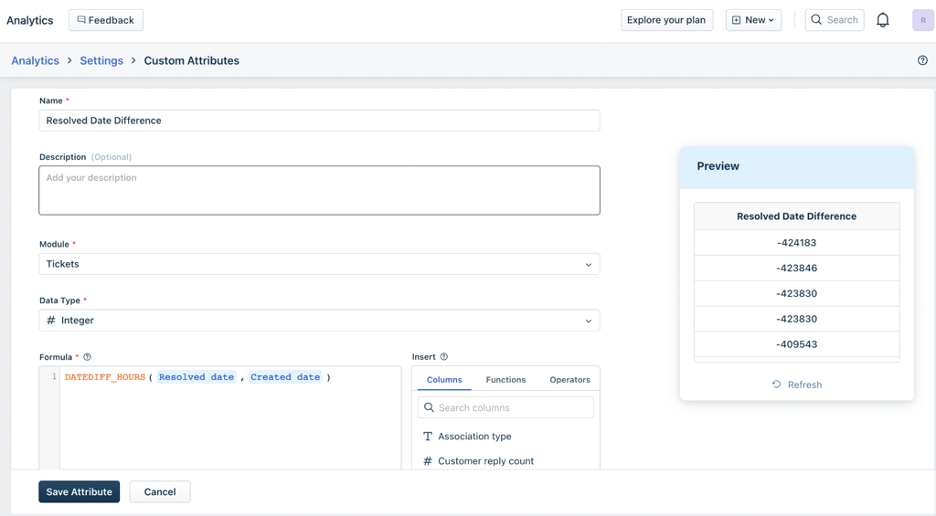You can create attributes of your choice and use them in filters or group-by to further organise or drill down your data using Custom Attributes.

• Click the Settings icon on the top-right corner• Select “Custom Attributes” from the side menu• Click on “Create Attribute” in the top right corner to start creating your custom metrics by using a combination of functions or operators.• Click “Save Attribute” once done
• Use this custom attribute to view the metrics the way you would like to

## Create your own Attributes and Group by

You can use the following functions to create your own Attributes and group-by.

 COMMAND MEANING Function - Date fx DATEDIFF_SECONDS Returns the difference between X and Y in seconds, X being the later date and Y being the earlier date fx DATEDIFF_MINUTES Returns the difference between X and Y in minutes, X being the later date and Y being the earlier date fx DATEDIFF_HOURS Returns the difference between X and Y in hours, X being the later date and Y being the earlier date fx DATEDIFF_DAYS Returns the difference between X and Y in days, X being the later date and Y being the earlier date fx DATEDIFF_WEEKS Returns the difference between X and Y in weeks, X being the later date and Y being the earlier date fx DATEDIFF_MONTHS Returns the difference between X and Y in months, X being the later date and Y being the earlier date fx DATEDIFF_YEARS Returns the difference between X and Y in years, X being the later date and Y being the earlier date fx SECOND Returns X with seconds as the unit fx MINUTE Returns X with minutes as the unit fx HOUR Returns X with hours as the unit fx DAY Returns X with days as the unit fx MONTH Returns X with months as the unit fx YEAR Returns X with years as the unit Function - Number fx CEIL Returns the least integer greater than or equal to X Example CEIL (2.5) is 3 fx FLOOR Returns the largest integer lesser than or equal to X Example FLOOR (2.5) is 2 fx ROUND Round the number up to the nearest integer value. Example ROUND (2.5) is 3 fx EXP Returns the value of e (Euler's number) to the power of X Example EXP (2) is 7.38906 fx GREATEST Returns greatest value among all fields Example GREATEST (1, 2, 3) returns 3 fx LEAST Returns least value among all fields Example LEAST (1, 2, 3) returns 1 fx LN Returns the value of natural logarithm function of X (base e logarithm of X) Example LN (2) is 0.6931471805599453 fx POWER Returns the value of X to the power of Y Example POWER (2, 3) will return 8 fx SQRT Returns the value of the square root of X. Example SQRT (4) will return 2 Function - String fx CONCAT Returns a string that is basically X1, X2, X3 strung together. Example CONCAT (Down, To, Boogie) will return 'DownToBoogie' as a value fx LEFT Returns a string that starts from a number of characters (n) from the beginning of X. Example LEFT (STRING, 1) will return 'TRING' fx RIGHT Returns a string that starts from a number of characters (n) from the end of X. Example RIGHT (STRING, 1) will return G fx LENGTH Returns the number of characters in X Example LENGTH (STRING) will return 5 fx LTRIM Returns the string X with leading spaces removed Example LTRIM ( STRING) will return 'STRING' fx RTRIM Returns the string X with trailing spaces removedExample RTRIM (STRING ) will return 'STRING' fx LPAD Returns the string with empty leading space padding on the left, based on the given length. Example LPAD(STRING, 2) will return ' STRING' fx RPAD Returns the string with empty trailing space padding to the right, based on the given length. Example RPAD (STRING, 2) will return 'STRING ' fx REPEAT Returns the string X repeated n times. Example REPEAT (STRING, 2) will return 'STRINGSTRING' fx REVERSE Returns the string X in reverse. Example REVERSE (STRING) will return 'GNIRTS' fx SUBSTRING Returns a substring of X, beginning from position 'n' - counted from left to right. Example SUBSTRING (STRING, 2) will return 'RING' fx TRIM Returns X with leading and trailing whitespace removed Example TRIM ( STRING ) will return 'STRING' Function - Logical fx BETWEEN Returns 1 if X is between Y and Z Example BETWEEN (2, 1, 3) will return 1 fx NOTBETWEEN Returns 1 if X is not between Y and Z Example NOTBETWEEN (1, 2, 3) will return 1 fx ISNULL Returns 1 if X is null, else 0 Example ISNULL (CLOSE DATE) fx ISNOTNULL Returns 1 if X is not null, else 0 Example ISNOTNULL (CLOSE DATE) Function - Operators + Addition - Subtraction * Multiplication / Division % Modular Division

## Sample metrics for you to get started:

Defining a custom resolution time which is the time taken from the ‘assigned’ stage to the ‘resolved’ stage. Ticket resolution by the bot is L0 and we do not want to take that into consideration.

Formula: datediff_hours(resolved date, created date)